# detectdrift

Detect drifts between baseline and target data using permutation testing

Since R2022a

## Syntax

``DDiagnostics = detectdrift(Baseline,Target)``
``DDiagnostics = detectdrift(Baseline,Target,Name=Value)``

## Description

example

````DDiagnostics = detectdrift(Baseline,Target)` performs Permutation Testing to detect drift for each variable in the `Baseline` and `Target` data sets, and returns the results in `DDiagnostics`.`DDiagnostics` is a `DriftDiagnostics` object.```

example

````DDiagnostics = detectdrift(Baseline,Target,Name=Value)` specifies additional options using one or more of the name-value arguments. For example, you can specify the metrics to use for the variables or the maximum number of permutations.```

## Examples

collapse all

Generate baseline and target data with two variables, where the distribution parameters of the second variable change for the target data.

```rng('default') % For reproducibility baseline = [normrnd(0,1,100,1),wblrnd(1.1,1,100,1)]; target = [normrnd(0,1,100,1),wblrnd(1.2,2,100,1)];```

Compare the two data sets for any drift.

`DDiagnostics = detectdrift(baseline,target)`
```DDiagnostics = DriftDiagnostics VariableNames: ["x1" "x2"] CategoricalVariables: [] DriftStatus: ["Stable" "Drift"] PValues: [0.2850 0.0030] ConfidenceIntervals: [2×2 double] MultipleTestDriftStatus: "Drift" DriftThreshold: 0.0500 WarningThreshold: 0.1000 Properties, Methods ```

DDiagnostics is a `DriftDiagnostics` object. `detectdrift` displays some of the object properties.

Display the confidence intervals for the estimated p-values.

`DDiagnostics.ConfidenceIntervals`
```ans = 2×2 0.2572 0.0006 0.3141 0.0087 ```

For the first variable, the lower bound of the confidence interval for the estimated p-value is greater than the warning threshold value of 0.1. Therefore, `detectdrift` determines that the target data for the first variable is stable compared to the baseline data. For the second variable, the upper bound of the confidence interval for the estimated p-value is smaller than the drift threshold of 0.05. Therefore, the drift status for this variable is `Drift`, which indicates that `detectdrift` detects the shift in the distribution parameters.

`detectdrift` uses the default Bonferroni method for testing multiple hypotheses. The function first divides the warning and drift thresholds by the number of p-values, which in this case is two. Then the function determines if any p-value is still lower than either threshold. Here, the second p-value is still lower than the modified drift threshold, so the function sets the `MultipleTestDriftStatus` to `Drift` for the overall data.

Visualize the permutation results for both variables.

```tiledlayout(1,2); ax1 = nexttile; plotPermutationResults(DDiagnostics,ax1,Variable="x1") ax2 = nexttile; plotPermutationResults(DDiagnostics,ax2,Variable="x2")```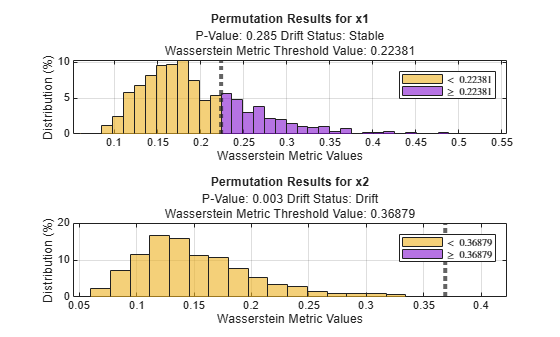Bars to the right of the dashed line show the metric values that are greater than the threshold, which is the initial metric value `detectdrift` computes using the baseline and target data for each variable. The amount of the bars greater than the threshold is much more for variable `x1`, which indicates that there is not a significant drift between the baseline and target data for this variable.

`load humanactivity`

For details on the data set, enter `Description` at the command line.

Assign the first 250 observations as baseline data and next 250 as target data.

```baseline = feat(1:250,:); target = feat(251:500,:);```

Test for drift on variables 5 to 10 using a warning threshold of 0.05 and a drift threshold of 0.01. All variables are continuous, so use the Kolmogorov-Smirnov metric for all variables. Specify the False Discovery Rate method as the multiple test correction.

```DDiagnostics = detectdrift(baseline(:,5:10),target(:,5:10),WarningThreshold=0.05, ... DriftThreshold=0.01,ContinuousMetric="ks",MultipleTestCorrection="fdr")```
```DDiagnostics = DriftDiagnostics VariableNames: ["x1" "x2" "x3" "x4" "x5" "x6"] CategoricalVariables: [] DriftStatus: ["Drift" "Drift" "Drift" "Stable" "Warning" "Drift"] PValues: [1.0000e-03 1.0000e-03 1.0000e-03 0.8810 0.0110 1.0000e-03] ConfidenceIntervals: [2×6 double] MultipleTestDriftStatus: "Drift" DriftThreshold: 0.0100 WarningThreshold: 0.0500 Properties, Methods ```

Display the confidence intervals for the estimated p-values.

`DDiagnostics.ConfidenceIntervals`
```ans = 2×6 0.0000 0.0000 0.0000 0.8593 0.0055 0.0000 0.0056 0.0056 0.0056 0.9004 0.0196 0.0056 ```

The lower confidence bound of the p-value for the 8th variable (variable name `x4`) is greater than the warning threshold. Therefore, `detectdrift` determines that the drift status for this variable is "`Stable"`. The upper confidence bound of the p-value for the 9th variable (variable name `x5`) is greater than the drift threshold, but lower than the warning threshold. Therefore, `detectdrift` determines that the drift status for this variable is "`Warning"`. Confidence intervals of all other variables are smaller than the drift threshold, so they have a drift status of "`Drift"`. Based on the False Discovery Rate method for multiple test correction, the function determines that the drift status for the overall data is "`Drift"`.

Visualize the p-values with the confidence intervals and corresponding drift status.

`plotDriftStatus(DDiagnostics)`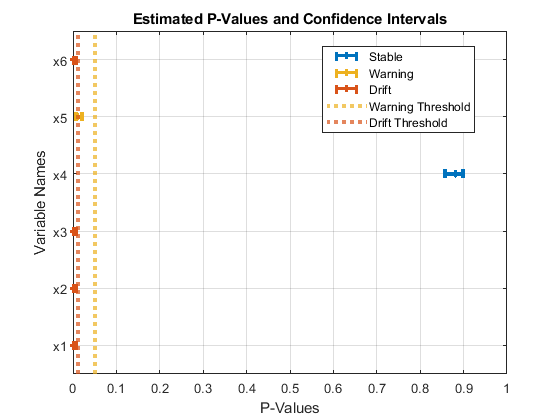The plot shows the estimated p-values with the confidence intervals against the warning and drift thresholds. The estimated p-value for variable `x4` and its confidence intervals are higher than the warning threshold. Therefore, the drift status for this variable is "Stable`"`. The upper confidence bound of the p-value for `x5` is greater than the drift threshold, but lower than the warning threshold. Therefore, the drift status for this variable is "`Warning"`. Confidence intervals of all other variables are smaller than the drift threshold, so they have a drift status of "`Drift"`.

`load NYCHousing2015`

The data set includes 10 variables with information on the sales of properties in New York City in 2015.

Remove outliers and convert the `datetime` array (`SALEDATE`) to the month numbers.

```idx = isoutlier(NYCHousing2015.SALEPRICE); NYCHousing2015(idx,:) = []; NYCHousing2015.SALEDATE = month(NYCHousing2015.SALEDATE);```

Define the baseline and target data as information on the sales made in January and July, respectively.

```tbl = NYCHousing2015; baseline = tbl(tbl.SALEDATE==1,:); target = tbl(tbl.SALEDATE==7,:);```

Shuffle the data.

```n = numel(baseline(:,1)); rng(1); % For reproducibility idx = randsample(n,n); baseline = baseline(idx,:); n = numel(target(:,1)); idx = randsample(n,n); target = target(idx,:);```

Test for potential drift between the baseline and target data. Specify the categorical variables and the metrics to use with each variable.

```DDiagnostics = detectdrift(baseline(1:1500,:),target(1:1500,:), ... VariableNames=["BOROUGH","BUILDINGCLASSCATEGORY","LANDSQUAREFEET","GROSSSQUAREFEET","SALEPRICE"], ... CategoricalVariables=["BOROUGH","BUILDINGCLASSCATEGORY"], ... Metrics=["Hellinger","Hellinger","ad","ks","energy"])```
```DDiagnostics = DriftDiagnostics VariableNames: ["BOROUGH" "BUILDINGCLASSCATEGORY" "LANDSQUAREFEET" "GROSSSQUAREFEET" "SALEPRICE"] CategoricalVariables: [1 2] DriftStatus: ["Drift" "Stable" "Drift" "Drift" "Drift"] PValues: [0.0260 0.1440 0.0070 0.0230 0.0110] ConfidenceIntervals: [2×5 double] MultipleTestDriftStatus: "Drift" DriftThreshold: 0.0500 WarningThreshold: 0.1000 Properties, Methods ```

`detectdrift` identifies drift between the baseline and target data for all variables except `BUILDINGCLASSCATEGORY`.

Display the confidence intervals for the estimated p-values.

`DDiagnostics.ConfidenceIntervals`
```ans = 2×5 0.0171 0.1228 0.0028 0.0146 0.0055 0.0379 0.1673 0.0144 0.0343 0.0196 ```

Plot a histogram for `SALEPRICE`.

`plotHistogram(DDiagnostics,Variable="SALEPRICE")`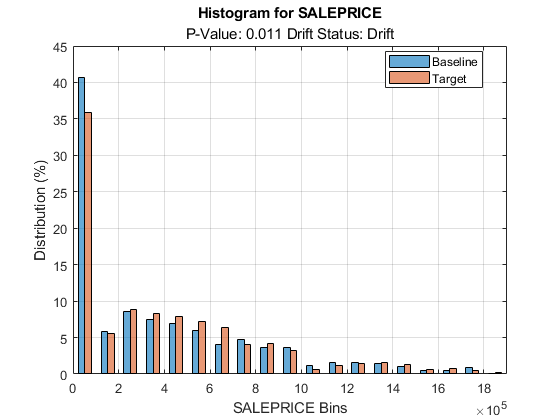The histogram shows the shift in the sale prices for the month of July compared to January.

Plot the empirical cumulative distribution function for the baseline and target data of `SALEPRICE`.

`plotEmpiricalCDF(DDiagnostics,Variable="SALEPRICE")`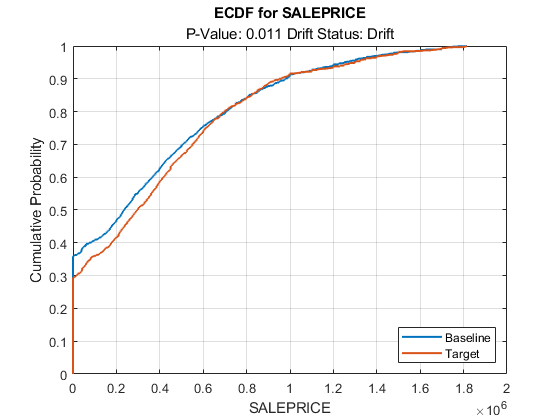Plot the permutation results for `SALEPRICE`.

`plotPermutationResults(DDiagnostics,Variable="SALEPRICE")`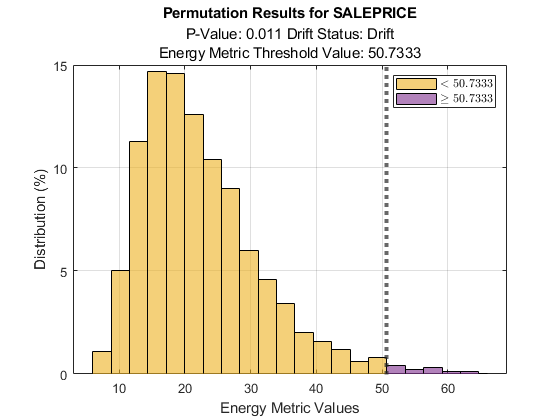Generate baseline and target data with three variables, where the distribution parameters of the second and third variables change for the target data.

```rng('default') % For reproducibility baseline = [normrnd(0,1,100,1),wblrnd(1.1,1,100,1),betarnd(1,2,100,1)]; target = [normrnd(0,1,100,1),wblrnd(1.2,2,100,1),betarnd(1.7,2.8,100,1)];```

Compute the initial metrics for all variables between the baseline and target data without estimating the p-values.

`DDiagnostics = detectdrift(baseline,target,EstimatePValues=false)`
```DDiagnostics = DriftDiagnostics VariableNames: ["x1" "x2" "x3"] CategoricalVariables: [] Metrics: ["Wasserstein" "Wasserstein" "Wasserstein"] MetricValues: [0.2022 0.3468 0.0559] Properties, Methods ```

`detectdrift` computes only the initial metric value for each variable using the baseline and target data. The properties associated with permutation testing and p-value estimation are either empty or contain `NaN`s.

`summary(DDiagnostics)`
``` MetricValue Metric ___________ _____________ x1 0.20215 "Wasserstein" x2 0.34676 "Wasserstein" x3 0.055922 "Wasserstein" ```

`summary` function displays only the initial metric value and the metric used for each specified variable.

`plotDriftStatus` and `plotPermutationResults` do not produce plots and return warning messages when you compute metrics without estimating p-values. `plotEmpiricalCDF` and `plotHistogram` plot the ecdf and the histogram, respectively, for the first variable by default. They both return `NaN` for the p-value and drift status associated with the variable.

`plotEmpiricalCDF(DDiagnostics)`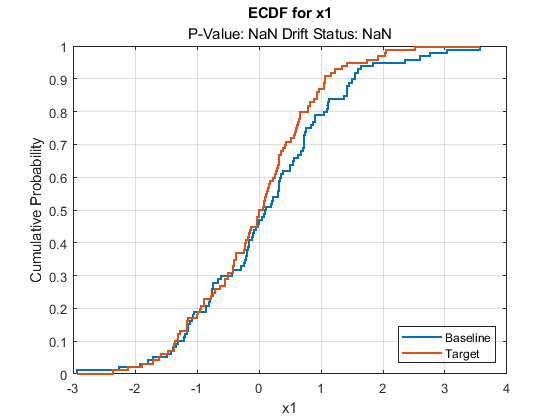`plotHistogram(DDiagnostics)`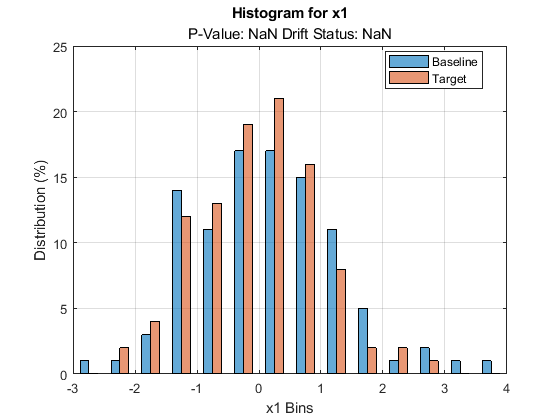## Input Arguments

collapse all

Baseline data, specified as a numeric array, categorical array, or table. `Baseline` and `Target` data must have the same data type. When the input data is a categorical array, `detectdrift` treats each column as an independent categorical variable.

Data Types: `single` | `double` | `categorical` | `table`

Target data, specified as a numeric array, categorical array, or table. `Baseline` and `Target` data must have the same data type. When the input data is a categorical array, `detectdrift` treats each column as an independent categorical variable.

Data Types: `single` | `double` | `categorical` | `table`

### Name-Value Arguments

Specify optional pairs of arguments as `Name1=Value1,...,NameN=ValueN`, where `Name` is the argument name and `Value` is the corresponding value. Name-value arguments must appear after other arguments, but the order of the pairs does not matter.

Example: `detectdrift(Baseline,Target,WarningThreshold=0.05,DriftThreshold=0.01,VariableNames=["Weight","MPG"],ContinuousMetrics="ad")` sets the warning threshold to 0.05 and drift threshold to 0.01, specifies Weight and MPG as the variables to test for drift detection, and Anderson-Darling as the metric to use in testing all continuous variables.

Variables to analyze for drift, specified as a string, array of unique strings, character vector, or cell array of character vectors.

Example: `VariableNames=["x1","x3"]`

Data Types: `string` | `char` | `cell`

List of categorical variables, specified as `"all"`, a string, array of unique strings, character vector, cell array of unique character vectors, vector of integer indices, or vector of logical indices.

`detectdrift` treats the following as categorical variables: `ordinal` or `nominal` data types, or the `categorical` data type with the ordinal indicator set to true as categorical variables.

Example: `CategoricalVariables="Zone"`

Data Types: `single` | `double` | `logical` | `string` | `cell`

Threshold for detecting drift, specified as a scalar value from 0 to 1.

`detectdrift` uses the drift threshold together with warning threshold to determine the drift status. The `DriftThreshold` value must be strictly lower than `WarningThreshold` value.

If the confidence interval for an estimated p-value is (Lower,Upper), then `detectdrift` determines the drift status as follows.

Drift StatusCondition
DriftUpper < `DriftThreshold`
Warning`DriftThreshold` < Lower < `WarningThreshold` or `DriftThreshold` < Upper < `WarningThreshold`
StableLower > `WarningThreshold`

Example: `DriftThreshold=0.01`

Data Types: `single` | `double`

Threshold for potential drift warning, specified as a scalar value between 0 and 1.

`detectdrift` uses the warning threshold together with drift threshold to determine the drift status. The `WarningThreshold` value must be strictly greater than the `DriftThreshold` value.

If the confidence interval for an estimated p-value is (Lower,Upper), then `detectdrift` determines the drift status as follows.

Drift StatusCondition
DriftUpper < `DriftThreshold`
Warning`DriftThreshold` < Lower < `WarningThreshold` or `DriftThreshold` < Upper < `WarningThreshold`
StableLower > `WarningThreshold`

Example: `WarningThreshold=0.05`

Data Types: `single` | `double`

Maximum number of permutations, specified as a positive integer value. `detectdrift` increases the number of trials for permutation logarithmically, according to a heuristic algorithm, until it determines the drift status or reaches `MaxNumPermutations`. If `detectdrift` cannot determine the drift status by the end of the maximum number of permutations, then it sets the drift status to `"Warning"`.

Example: `MaxNumPermutations=1500`

Data Types: `single` | `double`

Metrics used to detect drift for each variable, specified as one of the following:

• String, string vector, character vector, or cell array of character vectors representing one or more of the built-in metrics.

Built-in metrics for continuous variables

ValueDefinition
`"wasserstein"`Wasserstein
`"energy"`Energy
`"ks"`Kolmogorov-Smirnov
`"ad"`Anderson-Darling

Built-in metrics for categorical variables

ValueDefinition
`"tv"`Total Variation
`"psi"`Population Stability Index
`"hellinger"`Hellinger
`"chi2"`Chi-Square
`"bhattacharyya"`Bhattacharyya
• Function handle or a cell array of function handles. If you provide a function handle `FUN` as a metric, `detectdrift` calls it as:

`FUN(BaselineVariable,TargetVariable)`,

where `BaselineVariable` is the variable in `Baseline` and `TargetVariable` is the variable in `Target`. The output of `FUN` must be a scalar representing the metric value.

• Structure or a cell array of structures, where each structure contains a single field whose value is a function handle. If you pass a structure, `detectdrift` uses the field name as the metric name. If the function handle is anonymous, `detectdrift` names it `'CustomMetric_i'`, where i is the position of the variable in `Metrics`.

`Metrics` must contain one value for each variable in `VariableNames` and its size must be equal to the size of `VariableNames`.

If you specify metrics using `Metrics`, you cannot specify them using `ContinuousMetric` or `CategoricalMetric`.

Example: `Metrics=["wasserstein","psi","hellinger"]`

Data Types: `string` | `cell` | `function_handle` | `struct`

Metric for drift detection in continuous variables, specified as one of the following:

• String or a character vector representing one or more of the built-in metrics.

Built-in metrics for continuous variables

ValueDefinition
`"wasserstein"`Wasserstein
`"energy"`Energy
`"ks"`Kolmogorov-Smirnov
`"ad"`Anderson-Darling
• Function handle called as:

`FUN(BaselineVariable,TargetVariable)`,

where `BaselineVariable` is the variable in `Baseline` and `TargetVariable` is the variable in `Target`. The output of `FUN` must be a scalar representing the metric value.

If the function handle is not anonymous, `detectdrift` extracts the metric name from the provided function handle. If the function handle is anonymous, then it names the metric `'CustomContinuousMetric'`.

• Structure with a single field whose value is a function handle. In this case, `detectdrift` uses the field name as the metric name.

If you specify `ContinuousMetric`, then you cannot specify other metrics using `Metrics`.

Example: `ContinuousMetric="ks"`

Data Types: `string` | `char` | `function_handle` | `struct`

Metric for drift detection in categorical variables, specified as one of the following:

• String or a character vector representing one or more of the built-in metrics.

Built-in metrics for categorical variables

ValueDefinition
`"tv"`Total Variation
`"psi"`Population Stability Index
`"hellinger"`Hellinger
`"chi2"`Chi-Square
`"bhattacharyya"`Bhattacharyya
• Function handle called as follows:

`FUN(BaselineVariable,TargetVariable)`,

where `BaselineVariable` is the variable in `Baseline` and `TargetVariable` is the variable in `Target`. The output of `FUN` must be a scalar representing the metric value.

If the function handle is not anonymous, `detectdrift` extracts the metric name from the provided function handle. If the function handle is anonymous, then it names the metric `'CustomCategoricalMetric'`.

• Structure with a single field whose value is a function handle. In this case, `detectdrift` uses the field name as the metric name.

If you specify `CategoricalMetric`, then you cannot specify other metrics using `Metrics`.

Example: `CategoricalMetric="chi2"`

Data Types: `string` | `char` | `function_handle` | `struct`

Correction method for multiple hypothesis tests, specified as one of the following.

• `"bonferroni"` – Bonferroni correction. If k variables are specified for drift detection, `detectdrift` modifies the warning threshold and drift threshold by dividing each by k. Then, the function checks if any p-values are smaller than the modified threshold values to determine the drift status.

• `"fdr"` – False discovery rate (FDR) method. `detectdrift` uses the Benjamini-Hochberg procedure to compute the false discovery rate. If k variables are specified for drift detection, the FDR method takes these steps:

1. Rank the p-values corresponding to the specified variables.

2. Divide the ranks 1 to k by the number of variables k to obtain Q = [1/k, 2/k, 3/k ,…, k/k].

3. Modify the warning and drift thresholds for each sorted p-value by multiplying the initial warning and drift threshold values by the corresponding q value. For example, the modified warning threshold for rank 3 is (WarningThreshold)*3/k.

4. Check if any sorted p-values are smaller than the corresponding modified warning or drift thresholds to determine the drift status.

The multiple test correction methods provide a conservative estimate of the multivariable drift.

Example: `MultipleTestCorrection="fdr"`

Flag to run in parallel, specified as `true` or `false`. If you specify `UseParallel=true`, the `detectdrift` function executes `for`-loop iterations by using `parfor`. The loop runs in parallel when you have Parallel Computing Toolbox™.

Example: `UseParallel=true`

Indicator to estimate the p-values during permutation testing, specified as `true` or `false`. If you specify `EstimatePValues=false`, then `detectdrift` computes the metrics only.

Example: `EstimatePValues=false`

## Output Arguments

collapse all

Results of permutation testing for drift detection, returned as a `DriftDiagnostics` object. `detectdrift` displays the following properties.

Property NameDescription
`VariableNames`Variables analyzed for drift detection
`CategoricalVariables`Indices of categorical variables in the data
`DriftStatus`Drift status for each variable
`PValues`Estimated p-value for each variable
`ConfidenceIntervals`95% confidence interval bounds for the estimated p-values
`MultipleTestDriftStatus`Drift status for the overall data
`DriftThreshold`Threshold to determine the drift status
`WarningThreshold`Threshold to determine the warning status

For a full list of the properties and their descriptions, see the `DriftDiagnostics` reference page.

## Algorithms

collapse all

### Permutation Testing

`detectdrift` uses permutation testing  to determine the drift status for each variable in the `Baseline` data and its counterpart in the `Target` data. A permutation test is a nonparametric statistical significance test in which the function obtains distribution of a metric (test statistic) under the null hypothesis by computing the values of that metric under all possible rearrangements of a variable in `Baseline` and `Target`. Depending on the number of variables and observations, trying all possible permutations of a variable might be infeasible. Therefore `detectdrift` performs a sufficient number of permutations to obtain a good estimate of the metric for the variable.

Under null hypothesis (no drift), many values of the metric recorded during permutation testing can be as extreme as the initial test statistic. This suggests sufficiently high confidence that the observations of the specified variable in the baseline and target data come from the same distribution. Therefore, no evidence of drift is found, and `detectdrift` fails to reject the null hypothesis.

If the initial test statistic is identified as an outlier, then `detectdrift` rejects the null hypothesis. This suggest sufficiently high confidence that the observations of the specified variable in the baseline and target data come from different distributions. Therefore, drift is detected.

`detectdrift` takes these steps in permutation testing:

• For a given variable with m observations in the baseline data and n observations in the target data, `detectdrift` computes an initial value of the metric from the original data.

• The function then permutes the observations of the variable in the baseline and target data and separates them into two vectors with sizes m and n, respectively. Next, the function computes the same metric value. `detectdrift` repeats this step for `MaxNumPermutations` times to obtain a distribution of the specified metric.

• An estimate of the p-value is p = x/perm, where x is the number of times a metric value obtained from a permutation is greater than the value of the initial metric value, and perm is the number of permutations. With the binomial distribution assumption for x, `detectdrift` estimates the 95% confidence interval for the p-value by using `[~,CI] = binofit(x,perm,0.05)`.

Given the confidence intervals (Lower, Upper) of the p-values, `detectdrift` determines the drift status based on the following conditions.

Drift StatusCondition
DriftUpper < `DriftThreshold`
Warning`DriftThreshold` < Lower < `WarningThreshold` or `DriftThreshold` < Upper < `WarningThreshold`
StableLower > `WarningThreshold`

### Metrics

`detectdrift` uses the following metrics as test statistics in permutation testing for detecting drift between the baseline and target data.

Metrics for Continuous Variables

The `detectdrift` function first defines the following:

• ${E}_{b}\left(x\right)$ as the empirical cumulative distribution function (ecdf) of the baseline data over the common domain

• ${E}_{t}\left(x\right)$ as the ecdf of the target data over the common domain

• $D\left(x\right)$ as the joint ecdf of all data, and w as the difference between the edges of the bins

Next, `detectdrift` computes the metrics for continuous variables as follows:

• Wasserstein

`$W=\sum _{x}w*|{E}_{b}\left(x\right)-{E}_{t}\left(x\right)|$`
• Energy

`$En=\sqrt{2*\sum _{x}w*{|{E}_{b}\left(x\right)-{E}_{t}\left(x\right)|}^{2}}$`
• Kolmogorov-Smirnov

`$KS=\mathrm{max}|{E}_{b}\left(x\right)-{E}_{t}\left(x\right)|$`
• Anderson-Darling

`$AD={\sum _{x}\left(\frac{|{E}_{b}\left(x\right)-{E}_{t}\left(x\right)|}{\sqrt{\left(m+n\right)D\left(x\right)*\left(1-D\left(x\right)\right)}}\right)}^{2}$`

m and n are the number of observations in the baseline data and target data, respectively.

Metrics for Categorical Variables

The `detectdrift` function defines the following:

• ${H}_{b}\left(x\right)$ as the percentage of the baseline data in the bins determined by combining the baseline and target data (jointly considering them across the same domain)

• ${H}_{t}\left(x\right)$ as the percentage of the target data in the bins determined by combining the baseline and target data

Next, `detectdrift` computes the metrics for categorical variables as follows:

• Total Variation

`$TV=0.5*\sum _{x}|{H}_{b}\left(x\right)-{H}_{t}\left(x\right)|$`
• Population Stability Index

`$PSI=\mathrm{max}\left(0,\sum _{x}\mathrm{log}\left(\frac{{H}_{t}\left(x\right)}{{H}_{b}\left(x\right)}\right)\left({H}_{t}\left(x\right)-{H}_{b}\left(x\right)\right)\right)$`
• Chi-Square

`${\chi }^{2}=\sum _{x}\frac{{\left({H}_{t}\left(x\right)-{H}_{b}\left(x\right)\right)}^{2}}{{H}_{b}\left(x\right)}$`
• Bhattacharyya

`$B=\mathrm{max}\left(0,-\mathrm{log}\left(\mathrm{min}\left(1,\sum _{x}\sqrt{{H}_{b}\left(x\right)*{H}_{t}\left(x\right)}\right)\right)\right)$`
• Hellinger

`$H=\mathrm{max}\left(0,\sqrt{1-\left(\mathrm{min}\left(1,\sum _{x}\sqrt{{H}_{b}\left(x\right)*{H}_{t}\left(x\right)}\right)\right)}\right)$`

To handle empty bins (categories), `detectdrift` adds a 0.5 correction factor to histogram bin counts for each bin. This is equivalent to the assumption that the parameter p, which is the probability that the value of the variable is in that category, has the prior distribution Beta(0.5,0.5) (Jeffreys prior assumption for the distribution parameter).

 Hesterbeg,Tim, David S. Moore, Shaun Monaghan, Ashley Clipson, and Rachel Epstein. "Bootstrap Methods and Permutation Tests" in Introduction to the Practice of Statistics. 7th ed, W.H. Freeman, pp. 1–57, 2010.

 Benjamini, Yoav, and Yosef Hochberg. "Controlling the False Discovery Rate: A Practical and Powerful Approach to Multiple Testing." Journal of the Royal Statistical Society, Series B (Methodological). Vol. 57, No. 1, pp. 289-300, 1995.

 Villani, Cédric. Topics in Optimal Transportation. Graduate Studies in Mathematics. Vol. 58, American Mathematical Society, 2000.

 Deza, Elena, and Michel Marie Deza. Encyclopedia of Distances, Springer Berlin Heidelberg, 2009.